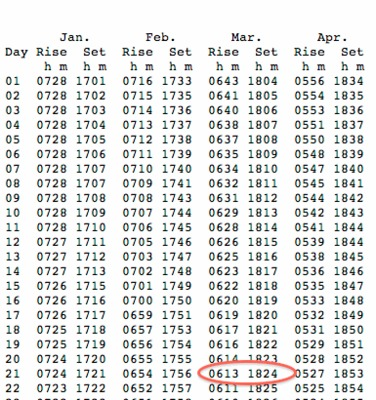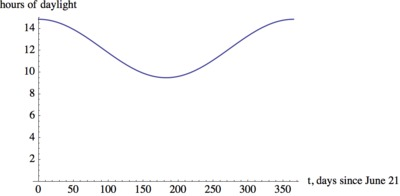# Hours of Daylight 1

Alignments to Content Standards: F-TF.B.5

In order to determine how effective solar panels would be at various times of the year it is important to know the amount of daylight at a given location. To be able to make easy calculations, it is often not sufficient to just have tables of data, which are readily available online.

Find an appropriate function that serves as a mathematical model for the hours of daylight in a location of your choice.

Use the model to find the hours of daylight on your birthday.

## IM Commentary

This task has a very short problem statement. The intention is that students think about a situation they have experienced and analyze it with mathematics. A natural solution is to model the hours of daylight with a sinusoidal function. Depending on how familiar students are with the modeling process, the instructor can concentrate on different aspects of of the modeling process. The CCSS introduction to modeling lists six steps that make up the modeling cycle:

(1) identifying variables in the situation and selecting those that represent essential features,

(2) formulating a model by creating and selecting geometric, graphical, tabular, algebraic, or statistical representations that describe relationships between the variables,

(3) analyzing and performing operations on these relationships to draw conclusions,

(4) interpreting the results of the mathematics in terms of the original situation,

(5) validating the conclusions by comparing them with the situation, and then either improving the model or, if it is acceptable,

(6) reporting on the conclusions and the reasoning behind them.

The solution to this task discusses many of theses steps. Most problems that are labeled as "modeling" problems in textbooks ask students to concentrate on (3) and (4). It is important to give students opportunities to engage in all aspects of modeling.

This task can be extended to a longer classroom activity by asking groups of students to find models for the hours of daylight in different locations. After students share their models they will discover that their functions have the same period and very similar midlines but different amplitudes. If one of the locations lies above the arctic circle or in the southern hemisphere, other interesting aspects of the model can be discussed.

It is interesting to note that the midline, i.e. the average amount of daylight, is not exactly 12 hours. This is because the sun is not just a point but a sphere with non-zero diameter and sunrise and sunset are measured as the time when the sun first appears and completely disappears.

## Solution

(1) Identify and select variables:

We would like to find a model for the hours of daylight in a specific location. First we pick a location: Rohnert Park, California (author's home town). Since the amount of daylight changes throughout the year, one variable to consider is time. At different days of the year we have different amounts of daylight, but every year, the cycle repeats itself (more or less), so we don't have to work with dates, just with days of the year. Daylight can be measured in hours and minutes, so this is another variable in the model.

(2) Formulate a model:

The amount of daylight is a periodic function of the day of the year. We will find a function $f(t)$ that gives the amount of daylight, in hours, on day $t$ of the year. We assume that every year follows the exact same pattern, which is a slight simplification of reality.

There are many websites that give sunrise/sunset data. For example

http://aa.usno.navy.mil/data/docs/RS_OneYear.php

A section of the data is shown here:In the full data table we have 365 days of sunrise and sunset data. We can use it to compute the amount of daylight during the day. Rather than computing the hours of daylight for every day, we only need two days if we choose a sinusoidal function to model the data - the longest and the shortest day.

The longest day is June 21 with sunrise at 0447 (4:47 am) and sunset at 1938 (7:38 pm). This gives 14 hours and 51 minutes of daylight. We convert this number to hours and get $14+51/60 = 14.85$ hours.

The shortest day is December 21 with sunrise at 7:24 and sunset at 16:54, which gives $9+30/60 = 9.5$ hours of daylight. Therefore we are looking for a sinusoidal function with amplitude equal $(14.85-9.48)/2 = 2.68$ hours and midline $(14.85+9.5)/2 = 12.175$ hours. The period of the function is 365. If we choose $t=0$ to correspond to June 21, the longest day of the year, we can use a cosine function without horizontal shift for our model:

$f(t) = 2.675\cos(2\pi/365 t)+12.175.$

A graph of the function shows all important features:(3)(4)(5) Use the model and see if the answer makes sense:

We are asked to use the model to find the hours of daylight on our birthday. My birthday is January 14, which is 207 days after the longest day of the year. Therefore,

$f(207) = 2.675\cos(2\pi/365 \cdot 207)+12.175 \approx 9.734.$

So on my birthday there are about 9 hours and 44 minutes of daylight. This number seems reasonable given that January 14 is a little more than 3 weeks after the shortest day of the year with 9.5 hours of daylight.